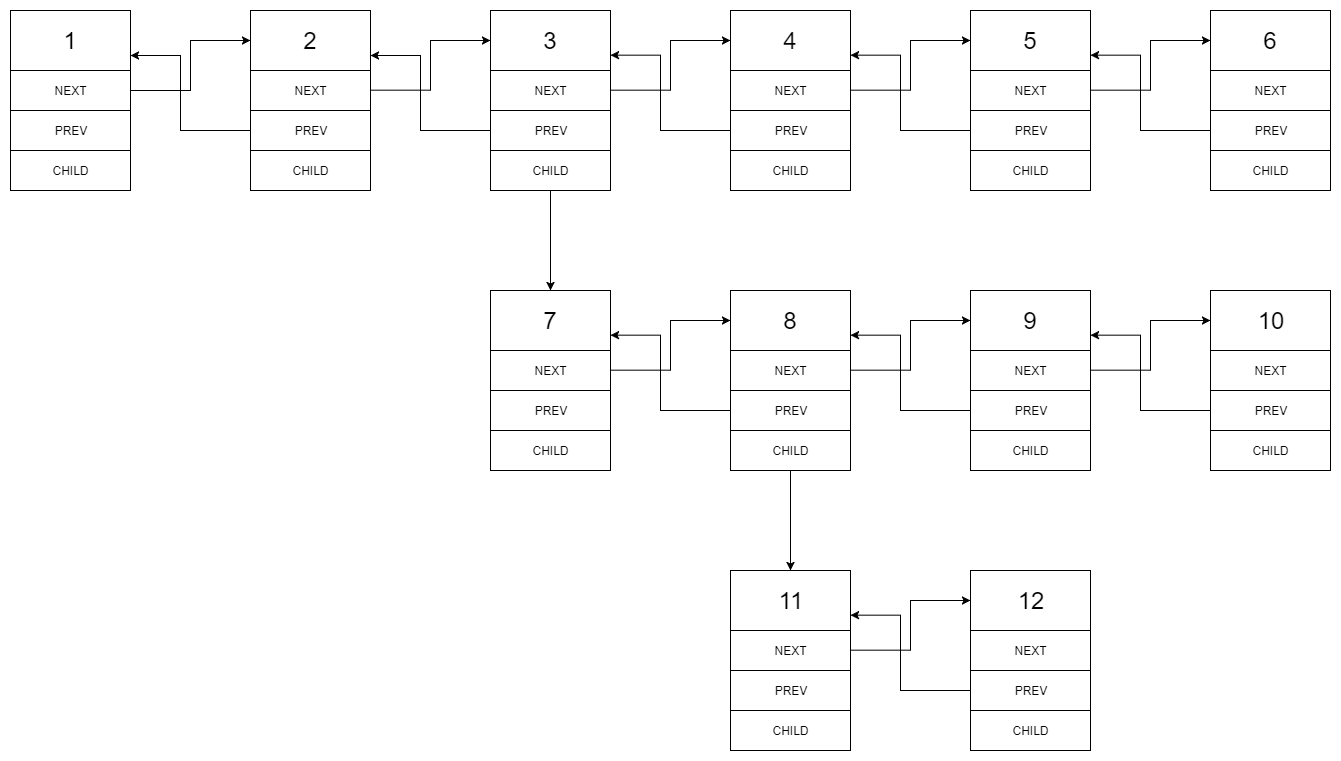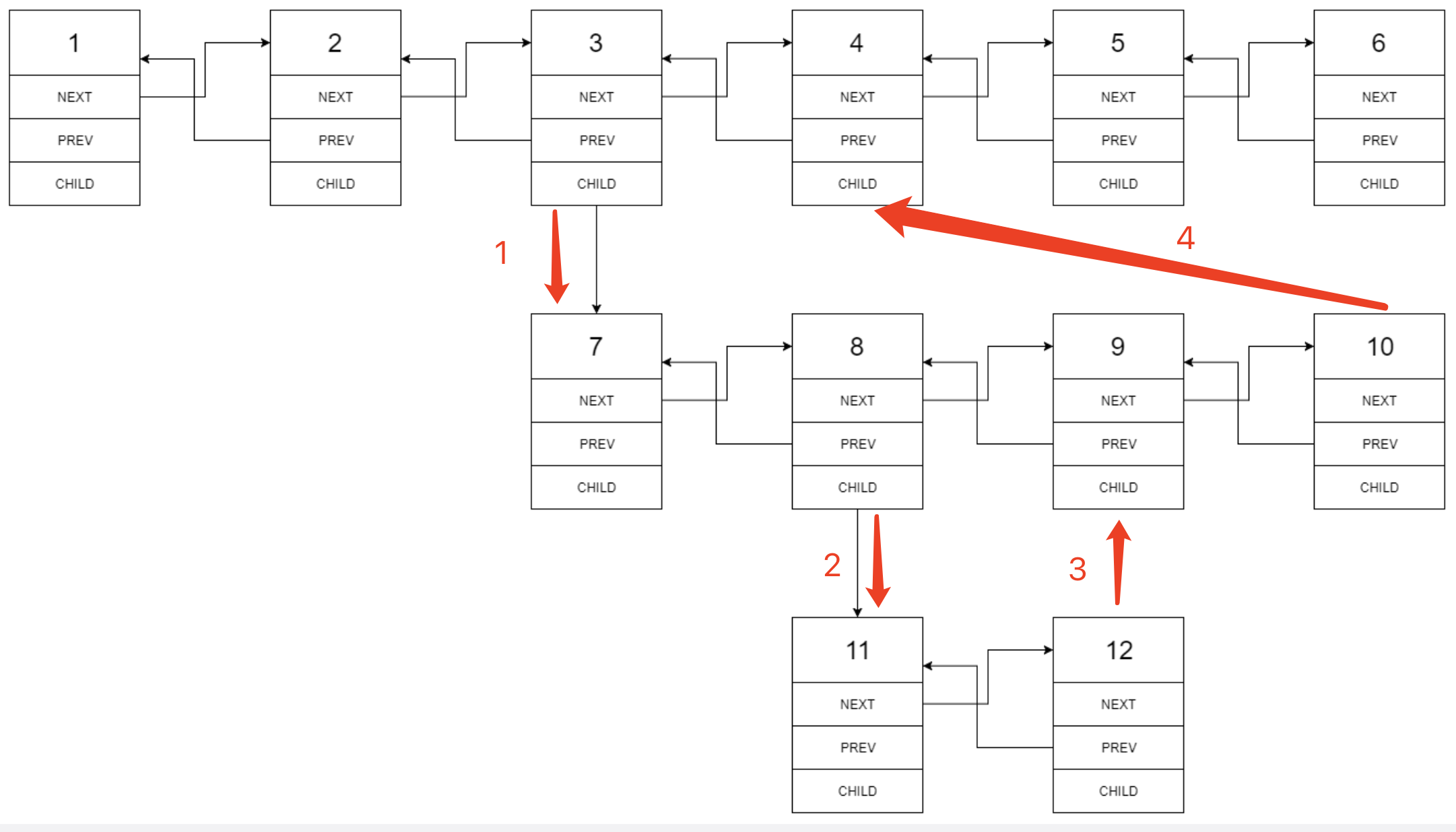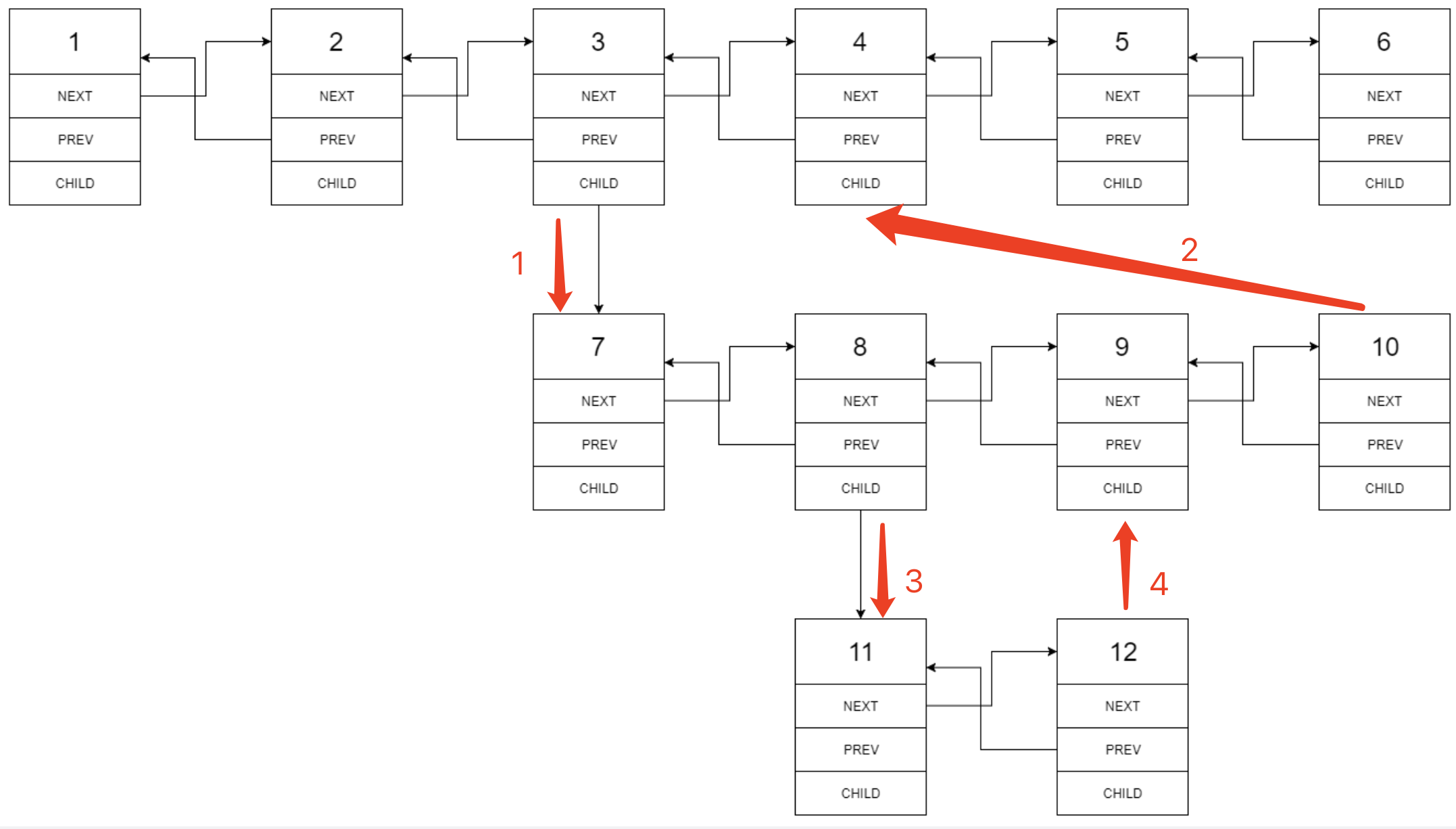# LC 430. 扁平化多级双向链表

### 题目描述### 递归

• 当前节点 $head$ 没有 $child$ 节点：直接让指针后即可，即 $head = head.next$；
• 当前节点 $head$ 有 $child$ 节点：将 $head.child$ 传入 flatten 函数递归处理，拿到普遍化后的头结点 $chead$，然后将 $head$ 和 $chead$ 建立“相邻”关系（注意要先存起来原本的 $tmp = head.next$ 以及将 $head.child$ 置空），然后继续往后处理，直到扁平化的 $chead$ 链表的尾部，将其与 $tmp$ 建立“相邻”关系。• 时间复杂度：最坏情况下，每个节点会被访问 $h$ 次（$h$ 为递归深度，最坏情况下 $h = n$）。整体复杂度为 $O(n^2)$
• 空间复杂度：最坏情况下所有节点都分布在 child 中，此时递归深度为 $n$。复杂度为 $O(n)$

### 递归（优化）• 时间复杂度：$O(n)$
• 空间复杂度：最坏情况下所有节点都分布在 child 中，此时递归深度为 $n$。复杂度为 $O(n)$

### 迭代• 时间复杂度：可以发现，迭代写法的扁平化过程并不与遍历方向保持一致（以段为单位进行扁平化，而非像递归那样总是往遍历方向进行扁平化），但每个节点被访问的次数仍为常数次。复杂度为 $O(n)$
• 空间复杂度：$O(1)$